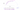## Polynomial Regression

Our hypothesis function need not be linear (a straight line) if that does not fit the data well. We can change the behavior or curve of our hypothesis function by making it a quadratic, cubic or square root function (or any other form).

For example, if our hypothesis function is $h_\theta(x) = \theta_0 + \theta_1 x_1$ then we can create additional features based on $x_1$, to get the quadratic function $h_\theta(x) = \theta_0 + \theta_1 x_1 + \theta_2 x_1^2$ or the cubic function $h_\theta(x) = \theta_0 + \theta_1 x_1 + \theta_2 x_1^2 + \theta_3 x_1^3$

In the cubic version, we have created new features $x_2$ and $x_3$ where $x_2 = x^2_1$ and $x_3 = x^3_1$.To make it a square root function, we could do:
$h_\theta(x) = \theta_0 + \theta_1 x_1 + \theta_2 \sqrt{x_1}$One important thing to keep in mind is, if you choose your features this way then feature scaling becomes very important. Eg. if $x_1$ has range 1 - 1000 then range of $x^2_1$ becomes 1 - 1,000,000 and that of $x^3_1$ becomes 1 - $10^9$